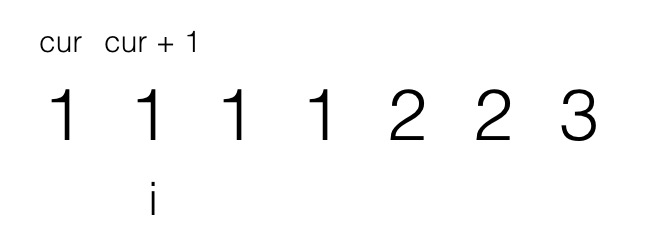Time Flying### 题目

不要使用额外的数组空间, 你必须在原地修改输入数组并在使用 O(1) 额外空间的条件下完成

给定数组 nums = [1,1,2],

给定 nums = [0,0,1,1,1,2,2,3,3,4],

// nums 是以“引用”方式传递的。也就是说, 不对实参做任何拷贝int len = removeDuplicates(nums);
// 在函数里修改输入数组对于调用者是可见的。// 根据你的函数返回的长度, 它会打印出数组中该长度范围内的所有元素。for (int i = 0; i < len; i++) {    print(nums[i]);}

### 解题

/** * @param {number[]} nums * @return {number} */var removeDuplicates = function (nums) {  const cacheObj = {}  let count = 0  while (count < nums.length) {    if (cacheObj[nums[count]]) {      nums.splice(count, 1)      count--    } else {      cacheObj[nums[count]] = nums[count].toString() // 防止 0 被判断为 false    }    count++  }  return nums.length}/** * @param {number[]} nums * @return {number} */var removeDuplicates = function (nums) {  if (nums.length <= 1) return nums.length  let cur = 0 // 新数组的下标  for (let i = 1; i < nums.length; i++) {    if (nums[i] !== nums[cur]) {      nums[cur + 1] = nums[i]      cur++    }  }  return cur + 1}

27、80、283。